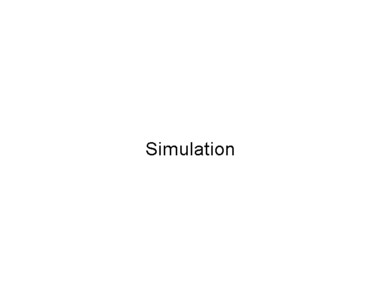# Articles

## Quantum Mechanical Simulations

Quantum mechanics describes microscopic phenomena on atomic length scales. It was developed in the twenties of the 20th century by Max Planck, Niels Bohr, Erwin Schrödinger, Werner Heisenberg and others, when it was revealed that the behaviour of the microcosmos differs fundamentally from that of the macroscopic world. Two of the main characteristics of the microscopic world are, firstly, the indistinguishability of wave-like and particle-like behaviour of corpuscular phenomena. Depending on the experimental setup, particles, such as electrons, can be interpreted as point-like bodies or as waves. And, secondly, dynamical quantities of a bound system, such as energy or angular momentum, can only take on certain quantized values which are discrete multiples of a particular constant, the Planck's action quantum. By leaving behind the classical conceptions of the end of the 19th century, quantum theory could be formulated in such a way as to describe the new discoveries, and it was able to make predictions, which were subsequently corroborated by experiment.

Contrary to all classical theories describing particle behaviour, quantum mechanics is of probabilistic nature. Each state is described by a wave function, which represents the probability amplitude for all possible configurations of the state. The time development of these wave functions, on the other hand, is completely deterministic and follows a partial differential equation called Schrödinger equation. As a consequence of the probabilistic concept, it is no longer justified to speak of a system's "trajectory". Statements about quantum systems are purely statistical and are interpreted as the result of measurements of many identical systems.

Apart from very simple systems, Schrödinger's equation can only be solved numerically, i.e. with the use of computers. Up to the end of the nineties, one was mostly relied on expensive parallel computers with dozens of processors. Nowadays, many problems can even be solved on modern PCs relatively fast. Check out some typical – admittedly simple – two-dimensional quantum phenomena each calculated on an Intel Pentium III 650 MHz processor within a few hours.

### Double slit experiment

The double slit experiment was one of the triggers for the development of quantum theory. When a homogeneous electron beam, which has passed through a double slit, is captured on a screen behind, one sees an interference pattern with a sequence of intensity minima and maxima, quite analogous to the diffraction of electromagnetic waves. Obviously and in contradiction to classical expectations, particles exhibit attributes, which leave room for a wave interpretation. On the other hand, the screen can only ever detect proper particles hitting the screen one by one. Accordingly, at a sufficient small radiation time, the interference pattern is discontinuous and indeed results from the bounces of each single particle on the screen.

A satisfying non-contradictory interpretation of this phenomen is obtained, if the strictly classical distinction between corpuscular and wave-like phenomena is abandoned and replaced by the postulate that each particle is described by a wave function ψ(x,t), whose intensity |ψ(x,t)|^2 is a measure of the probability of finding the particle at time t at location x. This is the content of the statistical interpretation of the wave-particle duality.

In these simulations, you see the probability density of an electron wave packet propagating towards an infinitely large double slit from different view points.### Step potential

Imagine a tennis ball flowing with constant velocity towards a step potential of infinite width. If the energy of the potential's right half is smaller than that of the ball (1st case), the ball is decelerated at the step and moves on constantly with smaller velocity. If, on the other hand, the potential's energy is larger (2nd case), the ball is completely reflected; think, for example, of a stone wall.

By contrast, a quantum mechanical particle behaves very different. In the first case, two scenarios are possible: The particle can either be reflected, thus moving backwards with the same velocity, or it moves onwards with smaller velocity just like the ball. In the second case, the particle turns out to be completely reflected, but not until a long enough time. In the meantime, the probability of finding the particle behind the step is non-zero. This strange phenomenon is called tunnel effect.

The following simulations show the probability density of a quantum mechanical particle, say electron, for both cases. The step of the potential is visualized by a flat wall, whose drawn height has no physical meaning.### Free wave packet

If you place a tennis ball on the floor and go to sleep afterwards, you can be sure, that the ball will be right there the next day. Doing the same with a quantum mechanical particle, you'll find the particle with less but non-zero probability anywhere else. This is because quantum mechanical wave packets spread out with time.